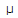# Electronics - Magnetism and Electromagnetism - Discussion

Discussion Forum : Magnetism and Electromagnetism - General Questions (Q.No. 4)
4.
How much flux is there in a magnetic field when its flux density is 5000T and its cross-sectional area is 300 mm2?
16.67 mWb
5.0Wb
3.0 mWb
1.5Wb
Explanation:
No answer description is available. Let's discuss.
Discussion:
9 comments Page 1 of 1.

Kelvin G said:   4 years ago
flux density = flux/area.
flux=flux density * -area,
= 5000uT * (300 * 10^-6)m2.
= 1.5.

Pankaj said:   4 years ago
B = fluxe*area.
B = 5000*10^-6,
A = 300÷10^3.

5000*300*10^-9 = 15 ÷ 10^4 = .0015web.

Ajith said:   8 years ago

Manoj said:   8 years ago
Flux = Flux density*area.

Flux = 5000*10^-6*300*10^-6 = 1.5 micro weber.

Luv said:   9 years ago
Given B = 5000 micro Tesla = 50 Gauss.
A = 300mm^2 = 3*10^(-4) m^2.

Flux = B.A = 50* .0003 = .015 Wb.

Teerthram meena said:   9 years ago
We now that magnetic flux is equal to product of flux density and cross sectional area so flux density is 1.5 micro Weber.

U is permeability,
fi=b*a;
b=flux density;
a=cross sec area
B=U*H
5000*300=1500000
=1.5UWb.